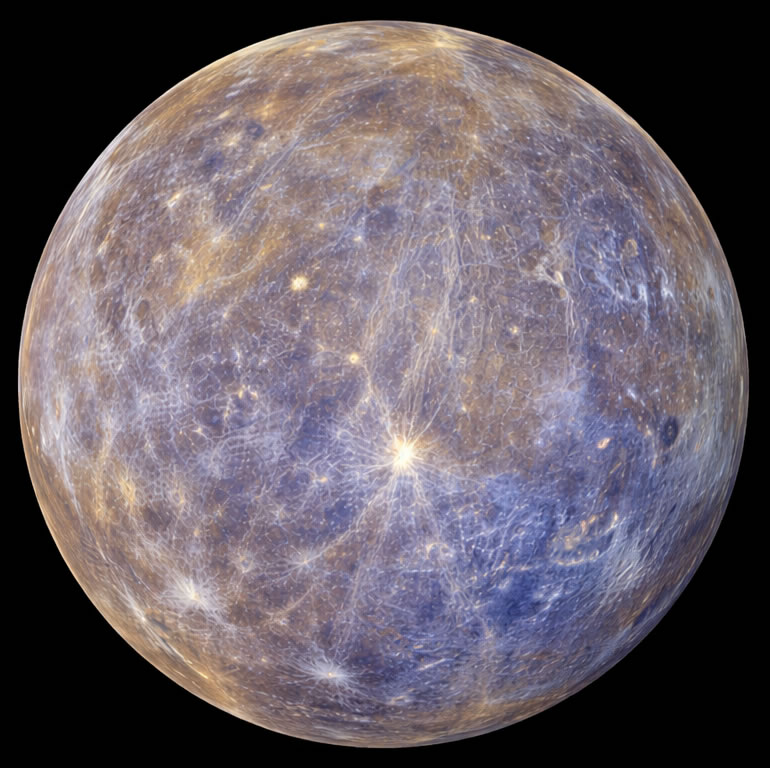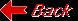Planet MercuryMass (kg) 3.303e+23 Mass (Earth = 1) 5.5271e-02 Equatorial radius (km) 2,439.7 Equatorial radius (Earth = 1) 3.8252e-01 Mean density (gm/cm^3) 5.42 Mean distance from the Sun (km) 57,910,000 Mean distance from the Sun (Earth = 1) 0.3871 Rotational period (days) 58.6462 Orbital period (days) 87.969 Mean orbital velocity (km/sec) 47.88 Equatorial surface gravity (m/sec^2) 2.78 Equatorial escape velocity (km/sec) 4.25 Mean surface temperature 179C Maximum surface temperature 427C Minimum surface temperature -173C Atmospheric composition: Helium 42%, Sodium 42%, Oxygen 15%, Other 1%